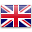# DC Motor (output)

Sort by:
##### 1.3 設計一個棲息地 Design a Habitat

STEM lessons + blocks

Students will gain understanding of how living things...##### 1.6 房子牢固性測試 Build it up, break it down

STEM lessons + blocks

Students will investigate how a house can be...##### 1.7 種子的傳播 Seed Dispersal

STEM lessons + blocks

Students will gain understanding of seed dispersal as...STEM lessons + blocks

Students will gain understanding of how technology can...##### 2.1 小夜燈 Night Light

STEM lessons + blocks

Students will gain understanding of how a product...##### 2.4 阻力與摩擦力 Resistance and Friction

STEM lessons + blocks

Students learn the concept of friction and the...##### 2.6 磁力 Magnetic Force

STEM lessons + blocks

Students will work collaboratively to investigate cause and...##### 2.7 打鼓模擬器 Drum Simulator

STEM lessons + blocks

Students will gain understanding of how the speed...##### 2.8 侵蝕 Erosion

STEM lessons + blocks

Students will gain understanding of water erosion and...##### 3.4 地震模擬器 Earthquake Simulator

STEM lessons + blocks

Students will gain understanding of what an earthquake...##### 3.5 火星探測號 Mars Rover

STEM lessons + blocks

Students will identify two possible solutions for designing...##### 3.6 燈塔 Lighthouse

STEM lessons + blocks

Students will investigate how light reflecting from objects...##### 3.7 信息的傳遞 SAM Insect

STEM lessons + blocks

Students will work collaboratively to investigate how information...##### 3.8 熔岩燈 SAM Lava Lamp

STEM lessons + blocks

Students will gain understanding of water erosion and...##### 4.2 地球和軌跡 Earth and Orbit

STEM lessons + blocks

Students will gain understanding of what an orbit...##### 4.3 黑夜與白晝 Night and Day

STEM lessons + blocks

Students will gain understanding of how the Earth...##### 4.6 牛頓第三運動定律 Every Action ....

STEM lessons + blocks

Students will gain understanding of Newton’s Third Law...##### 4.9 飲水機監控系統 Water Dispenser

STEM lessons + blocks

Students will gain understanding of the positive and...##### STEAM Course Kit - Team size

Bluetooth wireless sensor and output for coding

12 blocks, designed for 9-10 students. A STEAM...

HK\$ 3,680.00